06.04.2006, 07:54 #1 Moderator   Join Date: 09 2001 Location: South Korea, Gumi Posts: 7,699 Blog Entries: 16 Downloads: 102 Uploads: 34 Reputation: 561 | 6Knuth-Morris-Pratt algorithm Main featuresperforms the comparisons from left to right; preprocessing phase in O(m) space and time complexity; searching phase in O(n+m) time complexity (independent from the alphabet size); delay bounded by log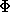(m) whereis the golden ratio (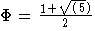). Description The design of the Knuth-Morris-Pratt algorithm follows a tight analysis of the Morris and Pratt algorithm. Let us look more closely at the Morris-Pratt algorithm. It is possible to improve the length of the shifts. Consider an attempt at a left position j, that is when the the window is positioned on the text factor y[j .. j+m-1]. Assume that the first mismatch occurs between x[i] and y[i+j] with 0 < i < m. Then, x[0 .. i-1] = y[j .. i+j-1] =u and a = x[i]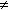y[i+j]=b. When shifting, it is reasonable to expect that a prefix v of the pattern matches some suffix of the portion u of the text. Moreover, if we want to avoid another immediate mismatch, the character following the prefix v in the pattern must be different from a. The longest such prefix v is called the tagged border of u (it occurs at both ends of u followed by different characters in x). This introduces the notation: let kmpNext[i] be the length of the longest border of x[0 .. i-1] followed by a character c different from x[i] and -1 if no such tagged border exits, for 0 < i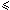m. Then, after a shift, the comparisons can resume between characters x[kmpNext[i]] and y[i+j] without missing any occurrence of x in y, and avoiding a backtrack on the text (see figure 7.1). The value of kmpNext is set to -1.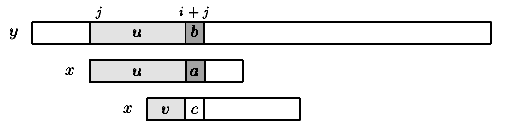Figure 7.1: Shift in the Knuth-Morris-Pratt algorithm (v border of u and cb). The table kmpNext can be computed in O(m) space and time before the searching phase, applying the same searching algorithm to the pattern itself, as if x=y. The searching phase can be performed in O(m+n) time. The Knuth-Morris-Pratt algorithm performs at most 2n-1 text character comparisons during the searching phase. The delay (maximal number of comparisons for a single text character) is bounded by log(m) whereis the golden ratio (). The C code void preKmp(char *x, int m, int kmpNext[]) { int i, j; i = 0; j = kmpNext = -1; while (i < m) { while (j > -1 && x[i] != x[j]) j = kmpNext[j]; i++; j++; if (x[i] == x[j]) kmpNext[i] = kmpNext[j]; else kmpNext[i] = j; } } void KMP(char *x, int m, char *y, int n) { int i, j, kmpNext[XSIZE]; /* Preprocessing */ preKmp(x, m, kmpNext); /* Searching */ i = j = 0; while (j < n) { while (i > -1 && x[i] != y[j]) i = kmpNext[i]; i++; j++; if (i >= m) { OUTPUT(j - i); i = kmpNext[i]; } } } The example Preprocessing phase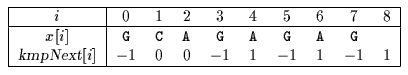The kmpNext table First attempt G C A T C G C A G A G A G T A T A C A G T A C G 1 2 3 4 G C A G A G A G Shift by: 4 (i-kmpNext[i]=3- -1) Second attempt G C A T C G C A G A G A G T A T A C A G T A C G 1 G C A G A G A G Shift by: 1 (i-kmpNext[i]=0- -1) Third attempt G C A T C G C A G A G A G T A T A C A G T A C G 1 2 3 4 5 6 7 8 G C A G A G A G Shift by: 7 (i-kmpNext[i]=8-1) Fourth attempt G C A T C G C A G A G A G T A T A C A G T A C G 1 G C A G A G A G Shift by: 1 (i-kmpNext[i]=1-0) Fifth attempt G C A T C G C A G A G A G T A T A C A G T A C G 1 G C A G A G A G Shift by: 1 (i-kmpNext[i]=0- -1) Sixth attempt G C A T C G C A G A G A G T A T A C A G T A C G 1 G C A G A G A G Shift by: 1 (i-kmpNext[i]=0- -1) Seventh attempt G C A T C G C A G A G A G T A T A C A G T A C G 1 G C A G A G A G Shift by: 1 (i-kmpNext[i]=0- -1) Eighth attempt G C A T C G C A G A G A G T A T A C A G T A C G 1 G C A G A G A G Shift by: 1 (i-kmpNext[i]=0- -1) The Knuth-Morris-Pratt algorithm performs 18 character comparisons on the example. http://www-igm.univ-mlv.fr/~lecroq/string/examples/exp8.html Share Share this post onDiggDel.icio.usTechnoratiTwitterThread ToolsShow Printable VersionEmail this Page
 На правах рекламы:Powered by vBulletin® Copyright ©2000 - 2020, Jelsoft Enterprises Ltd.